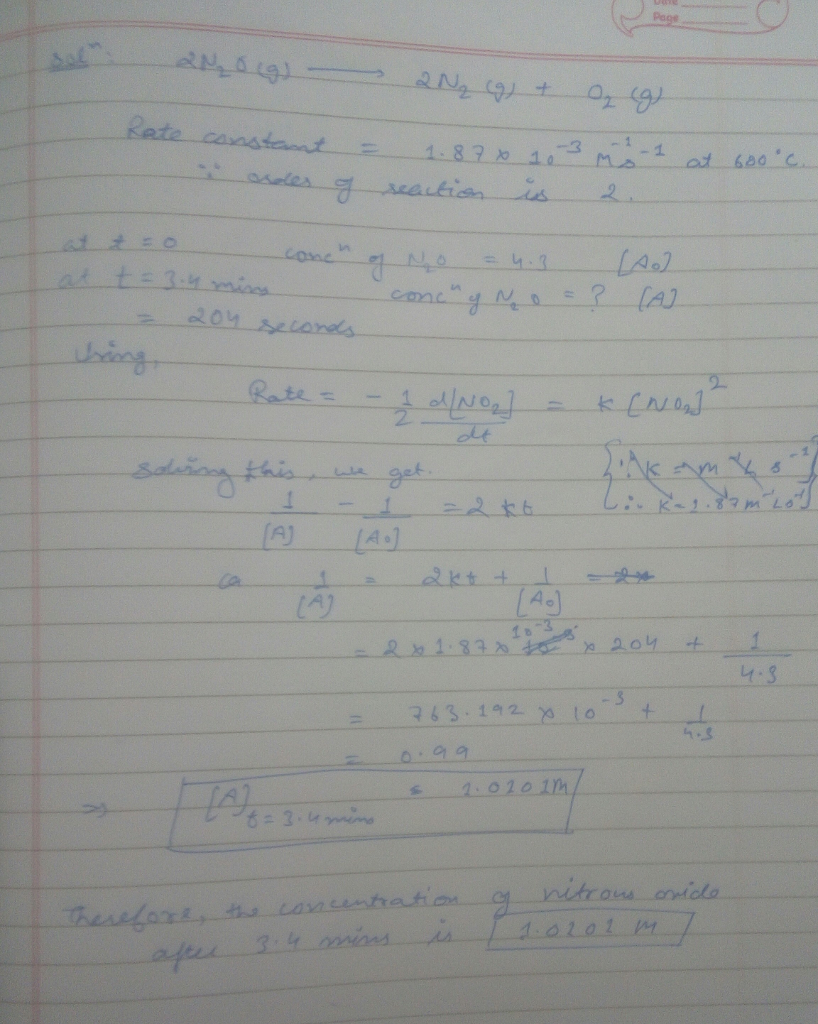# Homework Solution: Consider the decomposition of nitrous oxide to nitrogen and oxygen, 2N2O(g) → 2N2(g) +…

Consider the decomposition of nitrous oxide to nitrogen and oxygen, 2N2O(g) → 2N2(g) + O2(g) Without platinum as a catalyst, the reaction is 2nd order with a rate constant 1.87x10-3 M-1s-1 at 600°C. If the initial concentration of nitrous oxide was 4.3 M, what is the concentration of nitrogen gas after 3.4 mins? (Answer to 3 significant figures)